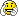# Gaussian Histogram

Hello Rooters,

I am new to Root and would like to seek your help.

I am trying to make a Gaussian histogram with the following code:

const Int_t NBINS = 6;
Double_t edges[NBINS + 1] = {-3.0,-2.0,-1.0,0.0,1.0,2.0,3.0};
TH1I *h=new TH1I(“h”, “Gaussian”, NBINS, -3.0, 3.0);
h->FillRandom(“gaus”,1000);
h->Fit(“gaus”);
h->Draw();

Here the histogram I am getting is by generating the random numbers. Could you please advice me how can I generate a histogram without doing FillRandom.

Thank you.

Regards,
Kajal

```{ const Int_t NBINS = 6; Double_t edges[NBINS + 1] = {-3.0, -2.0, -1.0, 0.0, 1.0, 2.0, 3.0}; TH1F *h = new TH1F("h", "Gaussian", NBINS, edges); for (Int_t i = 0; i < 1000; i++) { Double_t x = gRandom->Gaus(0.0, 1.5); // "x" Double_t w = 2.0 * gRandom->Rndm(); // "weight" h->Fill(x, w); // increment bin with abscissa "x" with a weight "w" } h->Fit("gaus"); h->Draw(); }```

Thank you Pepe Le Pew for your answer and code. I am obtaining a Gaussian histogram using it. But what I am looking for, is to make a Gaussian histogram without generating random numbers.

Probably by following a Gaussian function. The histogram obtained by generating random numbers is not symmetrical.

I hope I am clear with my query. I’ll be very glad to have any help and suggestion to get a symmetrical Gaussian histogram.

Thank you.

Regards,
Kajal

```{ const Int_t NBINS = 6; Double_t edges[NBINS + 1] = {-3.0, -2.0, -1.0, 0.0, 1.0, 2.0, 3.0}; TH1F *h = new TH1F("h", "Gaussian", NBINS, edges); TF1 *f = new TF1("f", "TMath::Gaus(x, 0.0, 1.5)", -3.0, 3.0); h->Eval(f); // h->Add(f, 1.0); h->Fit("gaus"); h->Draw(); }```

Thank you PePe Le Pew. It is working.Regards,
Kajal